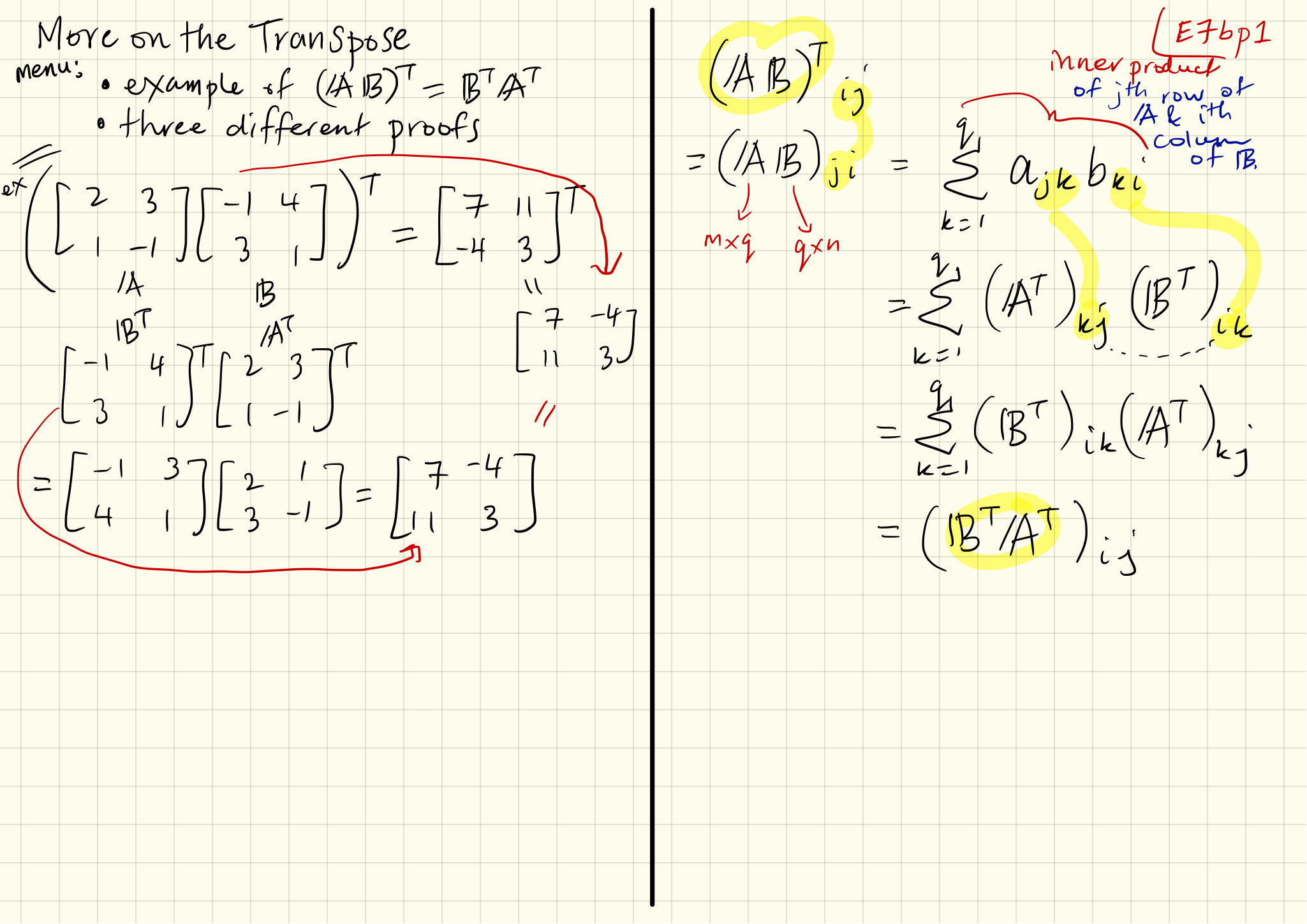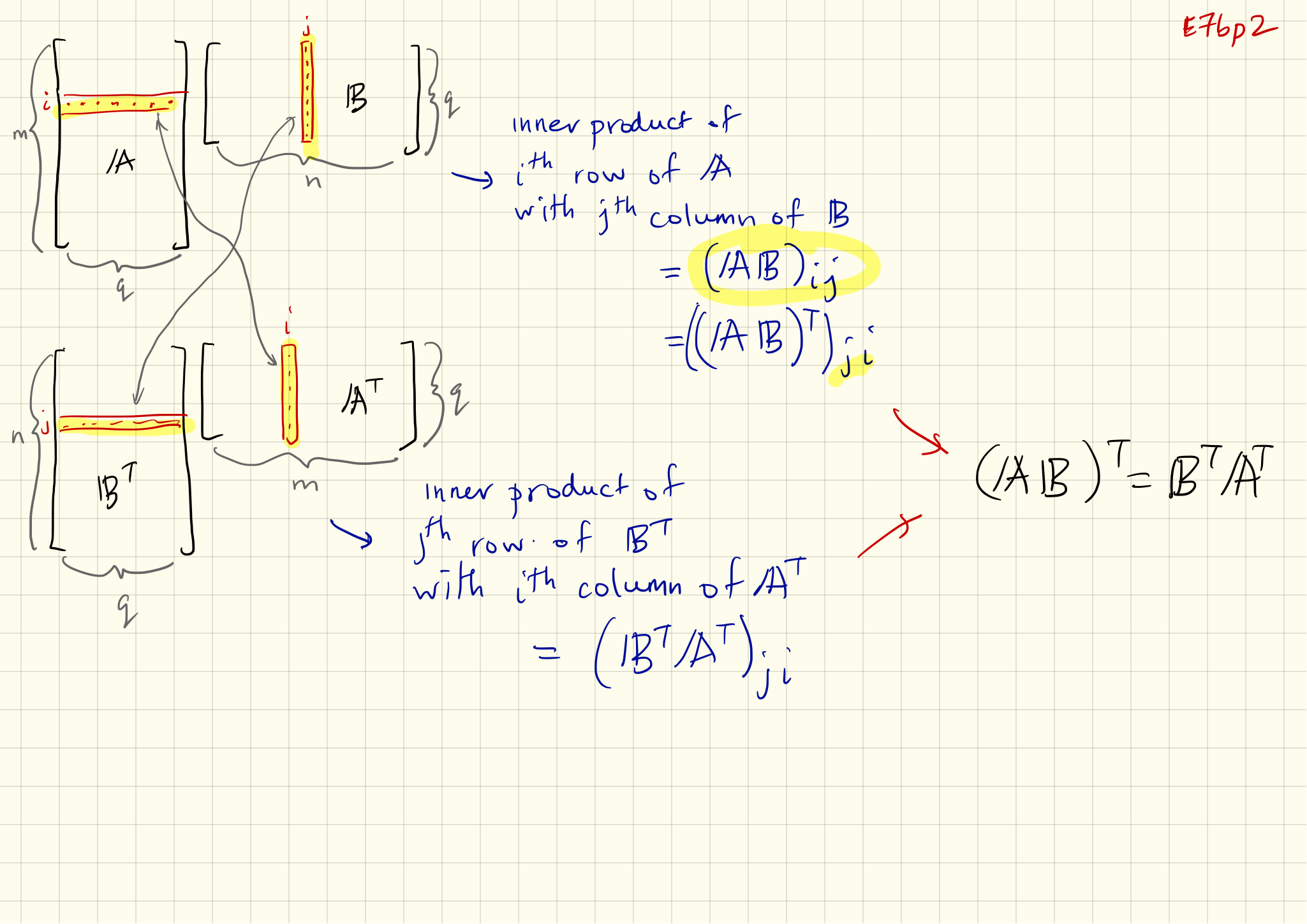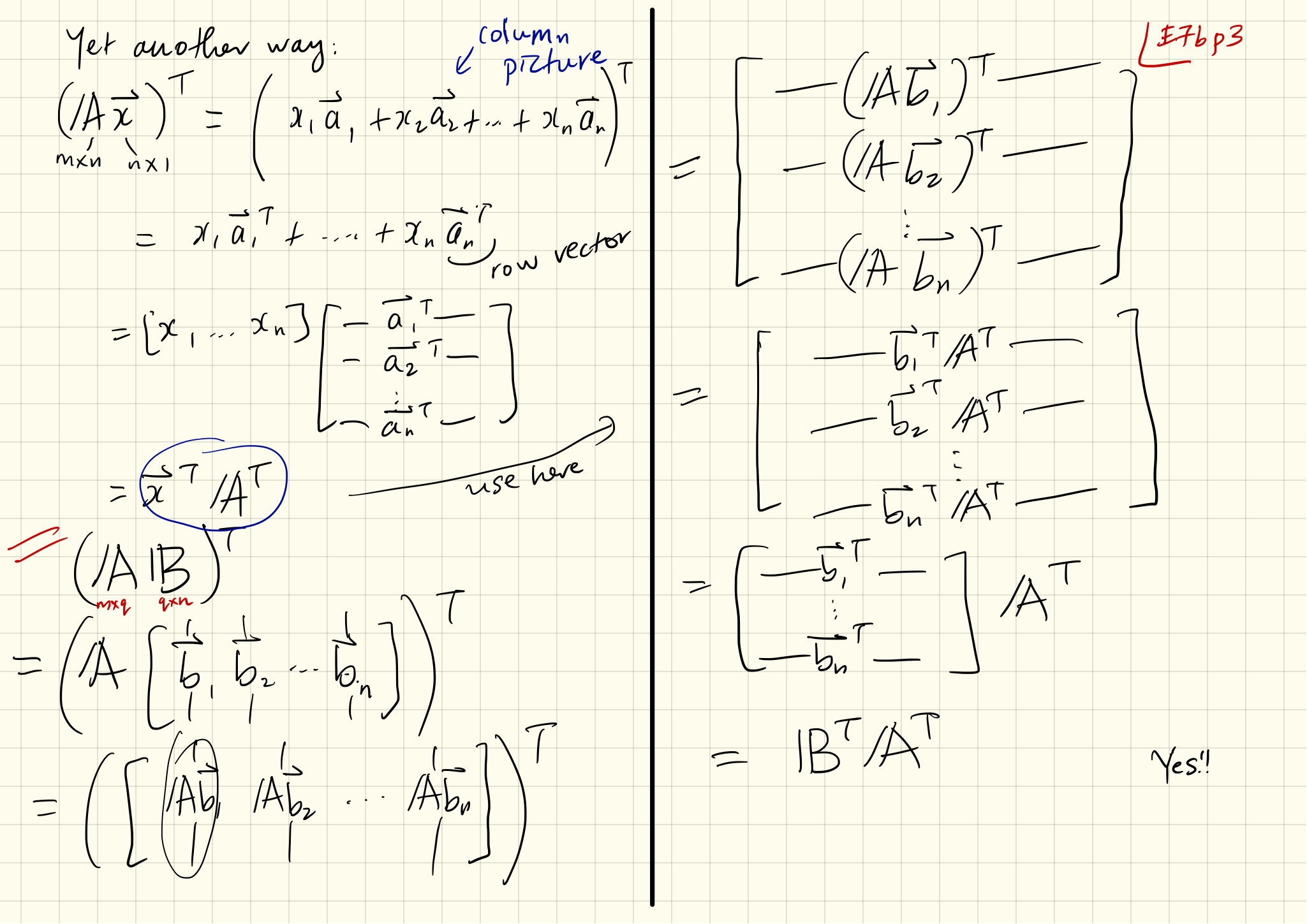Note: This is an archival, mostly functional site. All courses can be found here.

Note Set 07b: Three ways of understanding the tranpose's product rule

We give three explanations for why $(\mathbf{AB})^{\rm T} = \mathbf{B}^{\rm T} \mathbf{A}^{\rm T}$: Two algebraic ones, including advanced matrix wrangling, and a graphical version. Best consumed by 2016/09/16

•••